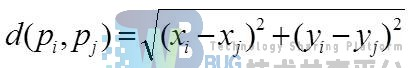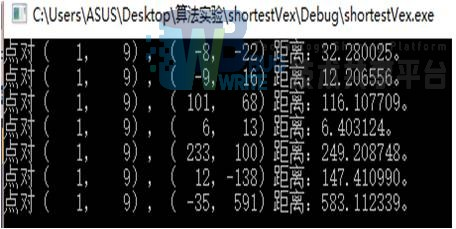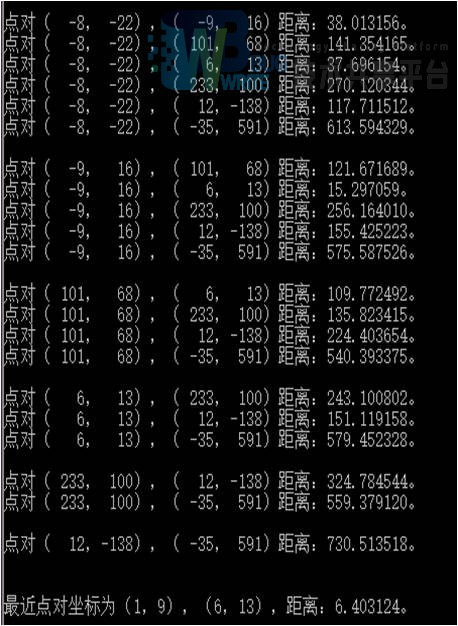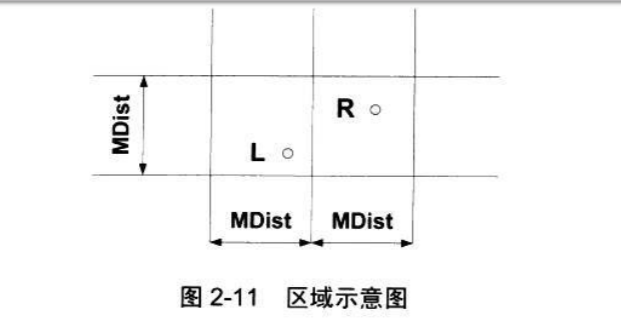• 1、实验题目最近点对问题：已知平面上分布着点集P中的n个点p1,p2,…pn，点i的坐标记为(xi,yi)，1≤i≤n。两点之间的距离取其欧式距离，记为：问题：找出一对距离最近的点。注：允许两个点位于同一个位置，此时两点...

1、实验题目

最近点对问题：

已知平面上分布着点集P中的n个点p1,p2,…pn，点i的坐标记为(xi,yi)，1≤i≤n。两点之间的距离取其欧式距离，记为：问题：找出一对距离最近的点。

注：允许两个点位于同一个位置，此时两点之间的距离为0。

2、设计思路

2.1 基本思路

由于不要求控制算法的时间复杂度，所以采用最简单直观的暴力求解法。

对于所有的n个点，计算任意两个点之间的距离，一边计算一边比较，遇到距离更近的点对则保存下来，这样可以在所有的距离中，选出最小的值，即为算法需要的结果。

2.2 数据结构设计

需要两个数组分别存放点的横坐标和纵坐标，数组下标代表点的序号，这里假定点的坐标都是整数，所以用int型数组。此外还需要一个浮点型变量用于计算两点间的距离，4个int型变量用于存放当前最近点对的坐标。

2.3 时间复杂度

将所有的点排成一排，从第一个点开始，依次求其后面的点与它的距离，要计算n-1次，第二个点需要计算n-2次……倒数第二个点计算1次，所以一共计算的次数为：

1+2+3+…+n-2+n-1=n(n-1)/2

因此算法时间复杂度为O(n)。

3、运行演示

测试用例以数组形式存放在代码中已经写好，不用手动输入，共8个点，坐标分别为：

(1，9)、(-8，-22)、(-9，16)、(101，68)、(6，13)、(233，100)、(12，-138)、(-35，591)，这些点覆盖了各大象限，距离也有近有远，足够验证算法的正确性，运行结果如图1-1和图1-2。遍历过程中每对点的距离都进行了输出，通过距离比较可以发现距离最近的两点是(1.9)和(-9，16)，距离是约为6.4。展开全文• 分支策略：(1)划分：将集合S分成两个子集S1和S2，根据平衡子问题原则，每个子集中大约有n/2个点，设集合S的最近点对是pi和pj(1<=i,j<=n)则有以下三种情况 1.pi∈S1,pj∈S12.pi∈S1,pj∈S23.pi∈S2, pj...

设p1=(x1,y1),p2=(x2,y2)...pn=(xn,yn)是平面n上n个点构成的集合S，最近对问你就是找出集合S中距离最近的点对。

分支策略：

(1)划分：将集合S分成两个子集S1和S2，根据平衡子问题原则，每个子集中大约有n/2个点，设集合S的最近点对是pi和pj(1<=i,j<=n)

则有以下三种情况 1.pi∈S1,pj∈S1

2.pi∈S1,pj∈S2

3.pi∈S2, pj∈S2

(2)求解子问题：对于划分阶段的情况1和2可递归求解

通过直线x=M(中位数),将空间划为两部分,xM分别求出左右最近点对距离，d1,d2,另d=min(d1,d2)

则，只需考虑3,即x-d和x+d的区域之间的最近点对，按照Y坐标对区域内点进行排序，如果一个点对的距离

小于d,他们一定在d*(2*d)的区域内。对于算法 nearest_pair( S,left,right)

1.预处理：对点集合S={(x1,y1),(x2,y2)......(xn,yn)}按照x坐标升序排列

2.如果n=2，则返回连点之间距离

3.m

计算{(x1,y1),(x2,y2).....(xm,ym)}之间的最近距离

计算{(xm,ym)(xm+1,ym+1)....(xn,yn)}之间的最近距离

d=min(d1,d2)

4.依次计算S[l...r]的最近点对(将集合按照y升序排列，考察y-s[m].y

l=min(i)| S[m]-S[i]

r=max(i)| S[i]-S[m]

// 实验四.cpp: 定义控制台应用程序的入口点。

//最近对问题

//2018.4.18

#include "stdafx.h"

#include

#include

#include

#include

#include

#include

using namespace std;

struct point {

double x;

double y;

}P;

double distance(point p1, point p2) {

return sqrt((p1.x - p2.x)*(p1.x - p2.x) + (p1.y - p2.y)*(p1.y - p2.y));

}

bool cmp1(point p1, point p2) {

return p1.x < p2.x;

}

bool cmp2(point p1, point p2) {

return p1.y < p2.y;

}

//蛮力法

double get_min(int n)

{

double min = sqrt((P.x - P.x)*(P.x - P.x) + (P.y - P.y)*(P.y - P.y));

for (int i = 0; i < n; i++) {

for (int j = i + 1; j < n; j++) {

double t = sqrt((P[i].x - P[j].x)*(P[i].x - P[j].x) + (P[i].y - P[j].y)*(P[i].y - P[j].y));

if (min>t)

min = t;

}

}

return min;

}

//分治法

double nearest_pair(point S[],int left,int right) {

cout << left << " " << right << endl;

if (right-left == 1) {

return distance(S[right], S[left]);

}

if (right - left == 2) {

double d1 = distance(S[right], S[left]);

double d2 = distance(S[right], S[right + 1]);

double d3 = distance(S[right + 1], S[left]);

d2 = min(d1, d2);

d3 = min(d2, d3);

return d3;

}

int m = (right+left) / 2;

double d1 = nearest_pair(S,left, m);

double d2 = nearest_pair(S, m+1,right);

//sort(S+right, S+left, cmp2);

double d = min(d1, d2);

int l = left, r = right;

while (S[l].x < S[m].x - d && l <= right);

l++;

while (S[r].x > S[m].x + d && r>=left)

r++;

sort(S + 1, S + r + 1, cmp2);

double d3;

for (int i = l; i <= r; i++) {

for (int j = i + 1; j <= r; j++) {

if (S[j].y - S[i].y >= d) {

break;

}

else {

d3 = distance(S[i], S[j]);

if (d3 < d)

d = d3;

}

}

}

return d;

}

int main()

{

int n;

cout << "Input n:";

cin >> n;

for (int i = 1; i <= n; i++) {

cout << "Input the " << i << "th number:";

cin >> P[i].x >> P[i].y;

}

sort(P + 1, P + n+1, cmp1);

for (int i = 1; i <= n; i++) {

cout << P[i].x << " " << P[i].y << endl;

}

double m = get_min(n);

cout << m << endl;

double m2 = nearest_pair(P, 1, n);

cout << m2 << endl;

system("pause");

return 0;

}

展开全文• (最近点对问题定义：已知上m个点的集合，找出对接近的一对点。)#include #include using namespace std;int closestPointPair(int * arr, int p, int q)//分治计算最近距离{if(p==q){return INT...

为最近对问题的一维版本设计一个直接基于分治技术的算法,并确定它的时间复杂度。假设输入的点是以升序保存在数组A中。(最近点对问题定义：已知上m个点的集合，找出对接近的一对点。)

#include

#include

using namespace std;

int closestPointPair(int * arr, int p, int q)//分治计算最近距离

{

if(p==q)

{

return INT_MAX;

}

else if(p

{

int m=(p+q)/2;

int Ldis = closestPointPair(arr, p, m);

int Rdis = closestPointPair(arr, m+1, q);

int dis = arr[m+1]-arr[m];

int Min = Ldis

Min = Min

return Min;

}

}

struct pointPair

{

int x;

int y;

};

struct pointPair closestPointpair(int *arr, int p, int q)//分治计算最近距离的点对

{

if(p==q)

{

struct pointPair pointpair = {INT_MIN,INT_MAX};

return pointpair;

}

else if(q-p==1)

{

struct pointPair pointpair = {arr[p], arr[q]};

return pointpair;

}

else

{

int m = (p+q)/2;

struct pointPair Lpointpair = closestPointpair(arr, p, m);

struct pointPair Rpointpair = closestPointpair(arr, m+1, q);

struct pointPair Cpointpair = {arr[m], arr[m+1]};

struct pointPair cpp = (Lpointpair.y-Lpointpair.x) < (Rpointpair.y-Rpointpair.x)?Lpointpair:Rpointpair;

cpp = (cpp.y-cpp.x) < (Cpointpair.y-Cpointpair.x)?cpp:Cpointpair;

return cpp;

}

};

int main()

{

int arr={1,4,6,8,10,11,15,17};

cout<

struct pointPair pp = closestPointpair(&arr, 0, 7);

cout<

return 0;

}

展开全文• 文章目录前言一、一维最近点对问题1、问题提出及代码求解2、时间复杂度分析二、二维最近点对问题1、问题提出及求解2、时间复杂度分析总结参考资料 前言 最近点对问题是分治法的典型应用案例，下面分别从一维和二维的...

前言

最近点对问题是分治法的典型应用案例，下面分别从一维和二维的角度给出了利用分治法求解最近点对的方法和代码，并且使用递归式和递归树的方法分析了时间复杂度。

一、一维最近点对问题

1、问题提出及代码求解

先输入一组点的个数，再输入数轴上这组点的坐标（整数），输出这组点之中最近的两点的距离。例：

输入5
1 3 5 6 8
输出1

代码如下：

#include<bits/stdc++.h>
using namespace std;

const int N = 1e6;
int A[N];//保存输入的数组

int closet_pot(int p, int q)
{
if(p == q)
return INFINITY;
if(p == q-1)
return A[q] - A[p];

int mid = (p+q)/2;
int a = closet_pot(p, mid);
int b = closet_pot(mid+1, q);

int Min = min(a, b);
Min = min(Min, A[mid+1] - A[mid]);
return Min;
}

int main()
{
int n;
cin>>n;
for(int i = 0; i < n; i++)
cin>>A[i];
sort(A, A+n);
cout<<closet_pot(0, n-1);
system("pause");
}

2、时间复杂度分析

按照分治法divide-conquer-merge的步骤分析，上述代码中的合并步骤：

Min = min(Min, A[mid+1] - A[mid]);

只有 O ( 1 ) O(1) 的时间复杂度，我们可以写出下列的递归式：
T ( n ) = { O ( 1 ) , n = 1 2 T ( n 2 ) + O ( 1 ) , n > 1 T(n) = \begin{cases} O(1), & n=1 \\[2ex] 2T(\frac{n}{2}) + O(1), & n>1 \end{cases}
使用递归树法进行分析：计算公式如下：
O ( 1 ) + O ( 2 ) + O ( 4 ) + O ( 8 ) ⋯ + O ( n ) ≈ O ( 2 n ) O(1)+O(2)+O(4)+O(8)\cdots+O(n) \approx O(2n)
所以使用分治法计算一维最近点对的时间复杂度大约为 O ( 2 n ) O(2n)

二、二维最近点对问题

1、问题提出及求解

下面是一道求二维最近点对的例题：Quoit Design。简单来说，就是把原先分布在数轴上的点分布在平面上，在这种情况下求最近点对。

求解代码参考了博客最近点对问题

/**
最近点对问题，时间复杂度为O(n*logn*logn)
*/
#include <iostream>
#include <cstdio>
#include <cstring>
#include <cmath>
#include <algorithm>
using namespace std;
const double INF = 1e20;
const int N = 100005;

struct Point
{
double x;
double y;
}point[N];
int n;
int tmpt[N];

bool cmpxy(const Point& a, const Point& b)
{
if(a.x != b.x)
return a.x < b.x;
return a.y < b.y;
}

bool cmpy(const int& a, const int& b)
{
return point[a].y < point[b].y;
}

double min(double a, double b)
{
return a < b ? a : b;
}

double dis(int i, int j)
{
return sqrt((point[i].x-point[j].x)*(point[i].x-point[j].x)
+ (point[i].y-point[j].y)*(point[i].y-point[j].y));
}

double Closest_Pair(int left, int right)
{
double d = INF;
if(left==right)
return d;
if(left + 1 == right)
return dis(left, right);
int mid = (left+right)>>1;
double d1 = Closest_Pair(left,mid);
double d2 = Closest_Pair(mid+1,right);
d = min(d1,d2);
int i,j,k=0;
//分离出宽度为d的区间
for(i = left; i <= right; i++)
{
if(fabs(point[mid].x-point[i].x) <= d)
tmpt[k++] = i;
}
sort(tmpt,tmpt+k,cmpy);
//线性扫描
for(i = 0; i < k; i++)
{
for(j = i+1; j < k && point[tmpt[j]].y-point[tmpt[i]].y<d; j++)
{
double d3 = dis(tmpt[i],tmpt[j]);
if(d > d3)
d = d3;
}
}
return d;
}

int main()
{
while(true)
{
scanf("%d",&n);
if(n==0)
break;
for(int i = 0; i < n; i++)
scanf("%lf %lf",&point[i].x,&point[i].y);
sort(point,point+n,cmpxy);
printf("%.2lf\n",Closest_Pair(0,n-1)/2);
}
return 0;
}

2、时间复杂度分析

关于合并（merge） 有更优化的做法，但是总的来说，时间复杂度是 O ( n ) O(n) ，可以写出下列递归式：
T ( n ) = { O ( 1 ) , n < 4 2 T ( n 2 ) + O ( n ) , n ≥ 4 T(n) = \begin{cases} O(1), & n<4 \\[2ex] 2T(\frac{n}{2}) + O(n), & n \geq 4 \end{cases}
得出下列的递归树：可以看到，一共有 log ⁡ 2 n \log_2{n} 层，每层时间复杂度为 O ( n ) O(n) ，总的时间复杂度为 O ( n l o g n ) O(nlogn)

总结

在最近点对问题上分治法得到了充分的应用，其实分治法不一定每次分为两边，可以分为三边甚至更多，比如改写二维点对代码中的 Closest_Pair​ 函数，每回分为3个子问题解决：

double Closest_Pair(int left, int right)
{
double d = INF;
if(left >= right)
return d;
if(left + 1 == right)
return dis(left, right);
int mid1 = (left+right)/3;
int mid2 = (left+right)*2/3;
double d1 = Closest_Pair(left,mid1);
double d2 = Closest_Pair(mid1+1,mid2);
double d3 = Closest_Pair(mid2+1, right);

d = min(d1,d2);
d = min(d, d3);

int i,j,k=0;
//分离出宽度为d的区间
for(i = left; i <= right; i++)
{
if(fabs(point[mid1].x-point[i].x) <= d)
tmpt[k++] = i;
}
sort(tmpt,tmpt+k,cmpy);
//线性扫描
for(i = 0; i < k; i++)
{
for(j = i+1; j < k; j++)
{
double d3 = dis(tmpt[i],tmpt[j]);
if(d > d3)
d = d3;
}
}

k = 0;
for(i = left; i <= right; i++)
{
if(fabs(point[mid2].x-point[i].x) <= d)
tmpt[k++] = i;
}
sort(tmpt,tmpt+k,cmpy);
//线性扫描
for(i = 0; i < k; i++)
{
for(j = i+1; j < k; j++)
{
double d3 = dis(tmpt[i],tmpt[j]);
if(d > d3)
d = d3;
}
}
return d;
}

在测试样例上都通过了，但可惜的是笔者在提交时遇到了超出内存限制的问题，未能解决。

如果分为3个子问题，递归式可以写成：
T ( n ) = { O ( 1 ) , n < 6 3 T ( n 3 ) + O ( c n ) , n ≥ 6 T(n) = \begin{cases} O(1), & n<6 \\[2ex] 3T(\frac{n}{3}) + O(cn), & n \geq 6 \end{cases}
相应的，递归树将有3个分叉，层数降低，但是每一层的时间复杂度比分为两个子问题增加了。

其中 c c 不太好确定，但是总的来说，和分为两个子问题的时间复杂度应该差别不大。

水平所限，难免纰漏，如有错误，欢迎指出。

参考资料

【1】最近点对问题

展开全文• 分治法求解最近点对问题一、实验目的与要求1、实验基本要求：2、实验亮点：二、实验内容与方法三、实验步骤与过程（一）一些准备工作1、实验流程2、数据生成与去除重复点（二）暴力穷举法1、算法描述2、时间复杂度...算法 数据结构 algorithm 分治算法
• （2）学会最近点对问题求解方法。 二、内容： 对于平面上给定的N个点，给出所有点对的最短距离，即，输入是平面上的N个点，输出是N点中具有最短距离的两点。 要求随机生成N个点的平面坐标，应用蛮力法编程计算出...算法 分治算法 数据结构
• 我们可以先从一维的寻找最近点寻找思路： 伪代码： NearestPointPairDim1(A[0,...,n-1]) { if (n = 1) return INF; if (n = 2) return d(A,A); d1 = NearestPointDim1(A[0,...,(n/2)-1]); d2 = ...
• 对于平面上的n个，请找出距离最近的两，给出这两的距离。 2.解析 当我们有两个的坐标时，我们很容易算出这两的距离。但是当的数量一旦多起来且我们想找出这些最近距离时，我们就需要以一个为中心...
• 最近对问题## 标题 在n个点中，距离最近的两个，在二维坐标平面中，两分别是a（x1，y1），b（x2，y2），则两距离是 d=sqrt((x1-x2)2+(y1-y2)2) 蛮力法，将平面内的n个，两两组队，计算最小距离，注意，这儿...算法 c++
• 在我试图找到每个位置的最近位置，并打印当前位置名称、到最近位置的距离和最近位置的名称。在存储在列0中的位置名纬度存储在第5列中经度存储在第8列中我已经试着解决这个问题，但是在几次迭代之后我得到了这个...
• 最近对问题要求在一个包含n个的集合中，找出距离最近的两个。 解析 求解最近对问题的蛮力算法是：分别计算每一对之间的距离，然后找出距离最小的一对。其中，为了避免同一对点计算两次距离，将范围压缩到...算法
• 这总是很有趣:)首先：Mohsen Nosratinia的回答是好的，只要您不需要... 这在极地地区将是一个更大的问题，因为那里的大的经度差异实际距离的影响较小 .球面坐标对于导航，绘图和类似的东西非常有用和实用 . 然而...
• ①数据插值可以根据有限个的取值状况，合理估算出附近其他的取值，从而节约大量的实验和测试资源，节省大量的人力、物力和财力。 ②数据插值能够根据已知数据推算未知数据，这使得人们解决问题的能力得到了拓展...matlab 数据插值
• 近期因为一些事情，有不少读者向笔者咨询chia矿池相关的信息，这一次为了方便大家，我专门写了一篇有关于chia矿池的相关消息的讲解，希望能够各位读者有所帮助。一共有以下几个方面： 1.积是什么以及如何计算？...
• 一段时间后，我在MATLAB Image Processing Toolbox中通过了imresize功能的代码，为图像的最近邻插值创建了一个简化版本。以下是如何应用于您的问题：%# Initializations:scale = [2 2]; %# The resolution scale ...
• 目录 1 KD树 1.1什么是KD树 1.2KD树的构建 1.3 KD树的插入 ... 特征匹配和数据库查、图像检索本质上是同一个问题，都可以归结为一个通过距离函数在高维矢量之间进行相似性检索的问题，如何快速而准确...
• 已经有一段时间没有更新博客了，因为最近变成了某个项目的负责人，就突然忙了起来，其实也就是一个接盘侠了。原本这个项目的负责人跑路了，而公司又没有其它合适的人选，所以只能让我当这个接盘侠。 刚接到这个项目...vue
• 韩信点兵算法

千次阅读 2020-12-23 11:58:10
最近，看书看到这个算法。很有意思。算法来源：话说有一次韩信带兵，人数在百人左右，然后它就那些士兵排队，3个人一行排的时候多了一个人，7个人一列排的时候少2个人，5个人排的时候刚刚好。刚开始碰到到这道题的...
• 最近很多微信用户问我微信定位是灰色的不开是怎么回事?该怎么解决问题呢?下面小编就带你了解一下微信定位是灰色的不开是怎么回事?该怎么解决问题呢?小伙伴们如果经常看微信朋友圈的话，肯定会看到不少的好友或者...
• Python快速查找每个站的最近的10个站

万次阅读 多人点赞 2021-01-08 23:07:05
作者：小小明，Pandas数据处理专家，致力于帮助无数数据从业者解决数据...保存结果整体完整代码求连接的基站不在最近6个基站内的采样数据读取将基站名称转换为索引获取最近的6个获取连接的基站不在最近6个基站内.
• 使用Python实现KNN算法解决简单分类问题 KNN分类 KNN算法属于监督学习算法，它可以解决分类... 我们将加入周围最近的 kkk 个找出来，加入的标签类别就是它周围这 kkk 个点中占比最多的那类标签。 第二种分类方机器学习 KNN算法 最近邻分类算法 分类算法 python
• 记录一个Unity打包包体过大的问题的解决方案和纹理相关知识梳理介绍。unity ios android
• 点击查看微信朋友圈好友设置仅展示最近3天动态是所有好友吗？还是针对某个人具体信息答：朋友圈显示三天是针对所有朋友，不能个别设置，个别设置只能设置让他不看你的朋友圈，以及陌生人可以或者不可以查看十张...
• 一、实验目的  1、熟悉并掌握MATLAB工具的使用...2、图像执行放大、缩小及旋转操作，分别采用最近邻插值、双线性插值及双三次插值方法实现，要求根据算法自己编写代码实现，并分析三种方法的优缺点。 （二）、相关知matlab 图像处理
• vue实现单点登录的N种方式

千次阅读 2021-08-04 06:11:37
最近项目停工了，RageFrame的学习暂时告一段落，这一篇给大家分享下有关单登录的相关知识，并提供一些demo给大家参考，希望想了解的朋友有一些帮助。 话不多说，先上原理(借鉴地址：...java cookie session jwt
• 浅谈最优化问题的KKT条件

千次阅读 2020-12-22 12:15:17
最近学习了最优化理论，正好学到了机器学习中支持向量机(Support Vector Machine)和最大熵模型(Maximum Entropy Model)中用到的KKT条件(Karush–Kuhn–Tucker conditions).之前看了一些相关书籍，发现KKT条件的证明...
• （15）创建种群个体与参考之间的 “映射关系”，其中 π ( s ) \pi(s) π(s)是距离种群个体最近的参考， d ( s ) d(\bf s) d(s)是参考与种群个体之间的距离； 计算小生境参考的出现次数（这个小生境是针对...NSGA-III 遗传算法 多目标进化算法 ENS
• 2k-最近邻法 2.1 算法概述 2.2kNN算法的一般流程 2.3距离公式 2.4k值的选择 2.5KNN特点 2.5.1特点 2.5.2KNN算法的优势和劣势 3距离加权最近邻算法 k-最近邻算法是基于实例的学习方法中最基本的，先介绍...机器学习 近邻算法 算法
• 过椭圆上任意一点的切线方程引发的思考与结论邓魁甲江西省赣州市第三中学 341000最近笔者在讲授高三第一轮复习时遇见复习资料上一个题目：过椭圆外一点 向椭圆 作切线，与椭圆切于 两，可知经过 两的直线的方程...
• ♥关于三角形平面上到三个顶点距离之和最小问题龚成通(山路水桥)答《爱问·知识人》友人问△ABC平面上到三个顶点距离之和PA+PB+PC最小的P，必满足：(1)当△ABC最大内角小于120°时，则P满足∠BPC=∠CPA=∠APB=120......